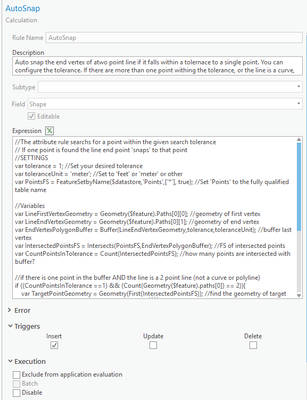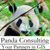# Using Attribute Rules to update the line geometry and 'Auto Snap' to a point if it falls within a specified tolerance

745
2
10-01-2021 07:21 AM
Labels (1)byEsri Regular Contributor
4 2 745

Problem:

When you enter COGO measurements to create a feature it will not snap to any other feature.

This can happen when you enter lot lines in a subdivision when you 'connect the dots' .

The Solution:

Disclaimer: this is R&D at this stage. If you are using true curves Arcade will densify them. So please don’t use on real data, this is not production-ready.”

Use attribute rule calculation to update the end vertex of a line if it falls within a specified tolerance to a point feature class.You can see that:

1. The entered COGO dimensions are preserved

2. While the line fell short it updated the end vertex to the point.

How this is implemented?

When creating a new line (Insert trigger event), the end vertex of the line is extracted and buffered with the specified tolerance.

That buffer is then intersected with the points.

The number of intersected points are counted - if there is exactly one point that intersects the buffer the geometry of the line (\$feature) is updated.

This attribute rule returns a geometry ('Polyline' method) that is calculated directly into the shape field.

Here is the expression:

``````//The attribute rule searchs for a point within the given search tolerance
// If one point is found the line end point 'snaps' to that point
//SETTINGS
var tolerance = 1; //Set your desired tolerance
var toleranceUnit = 'meter'; //Set to 'feet' or 'meter' or other
var PointsFS = FeatureSetbyName(\$datastore,'Points',['*'], true); //Set 'Points' to the fully qualified table name

//Variables
var LineFirstVertexGeometry = Geometry(\$feature).Paths; //geometry of first vertex
var LineEndVertexGeometry = Geometry(\$feature).Paths; //geometry of end vertex
var EndVertexPolygonBuffer = Buffer(LineEndVertexGeometry,tolerance,toleranceUnit); //buffer last vertex
var IntersectedPointsFS = Intersects(PointsFS,EndVertexPolygonBuffer); //FS of intersected points
var CountPointsInTolerance = Count(IntersectedPointsFS); //how many points are intersected with buffer?

//if there is one point in the buffer AND the line is a 2 point line (not a curve or polyline)
if ((CountPointsInTolerance ==1) && (Count(Geometry(\$feature).paths) == 2)){
var TargetPointGeometry = Geometry(First(IntersectedPointsFS)); //find the geometry of target point
var x1 = TEXT(LineFirstVertexGeometry.x); //start X coordinate
var y1 = TEXT(LineFirstVertexGeometry.y); //start Y coordinate
var x2 = TEXT(TargetPointGeometry.x); //target X coordinate
var y2 = TEXT(TargetPointGeometry.y); //target Y coordinate
var SR = Geometry(\$feature).spatialReference; //spatial reference of the line
var polylineJSON =  { //create a JSON representation of the updated line
"hasZ":true,
"paths":[[[x1,y1],[x2,y2]]],
"spatialReference":SR
};

return Polyline(polylineJSON); //return the Polyline to update the SHP field
}``````

Attribute rule setting:1. Do you find this useful?

2. Should it auto-snap to start/end vertices of other lines instead of the points?

3. Should we ship it with the parcel fabric

4. If you are using it, do you see undesired behavior?

If you deploy it with real data,  make sure to reduce the tolerance. It was set to one for testing/development purposes.byEsri Regular Contributor

@XanderBakker - please continue to share knowledge and samples. They are fantastic.New Contributor III

Amir,

This is very interesting and could solve some issues that we have experienced when building subdivisions. I will download the sample and test the code with some data.

Great idea.

Frank Conkling - Panda Consulting.